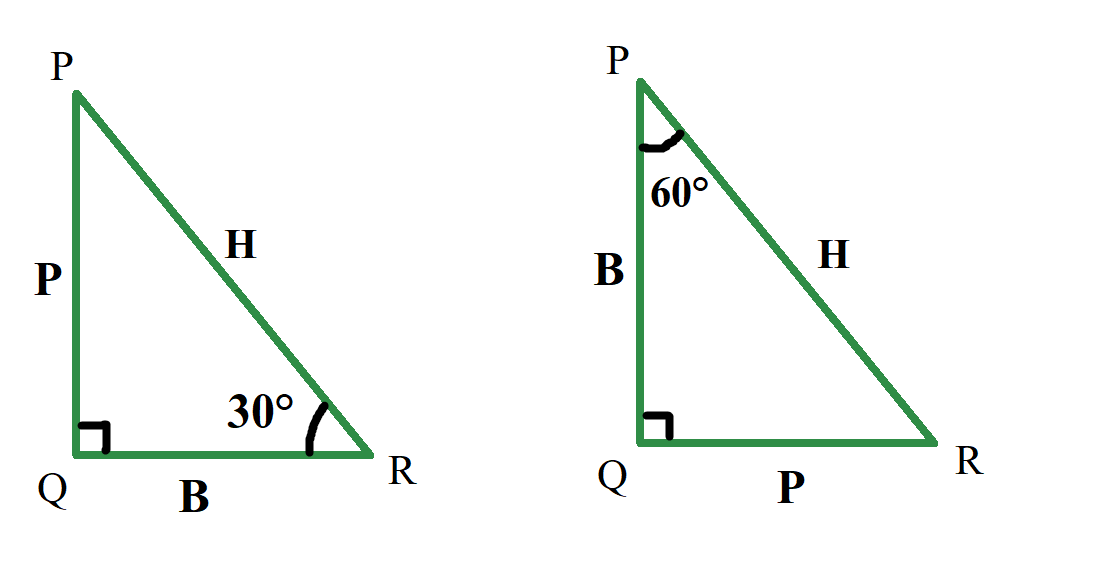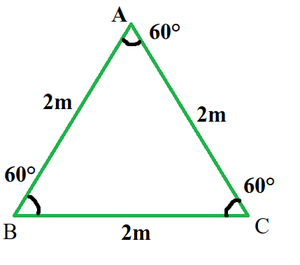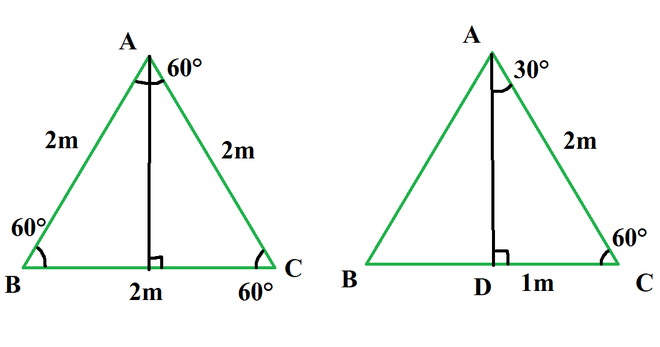Find the value of sin 30°.

• Difficulty Level : Medium
• Last Updated : 22 Sep, 2021

Trigonometry is the branch of mathematics that deals with the relationship of sides with angles in a triangle. With trigonometry finding out the heights of big mountains or towers is possible, also in astronomy, it is used to find the distance between stars or planets and is widely used in physics, architecture, and GPS navigation systems. Trigonometry is based on the principle that “If two triangles have the same set of angles then their sides are in the same ratio”. Side length can be different but side ratios are the same.

Trigonometric Functions

Trigonometric functions are also called circular functions or trigonometric ratios. The relationship of angles and sides is represented by these trigonometric functions. There are six trigonometric functions Sine, Cosine, Tangent, Cosecant, Secant, Cotangent. The sides representations for the six ratios are,

• sin A = Perpendicular / Hypotenuse
• cos A = Base / Hypotenuse
• tan A = Perpendicular / Base
• cot A = Base / Perpendicular
• sec A = Hypotenuse / Base
• cosec A = Hypotenuse / Perpendicular.

Here, A is the angle opposite to the perpendicular side. Let’s learn what the Perpendicular, base, and hypotenuse of a right-angled triangle are,

1. Perpendicular: The side in front of the angle is perpendicular. In this case, the side in front of 30 deg is called it’s perpendicular.
2. Base: A base is one of the sides which touches angle, but hypotenuse can never be considered as base.
3. Hypotenuse: It is a side opposite to 90°. it is the largest side.

Note: Perpendicular and base changes as angle changes. In a triangle, a side is perpendicular for an angle, but the same side is a base for another angle, but the hypotenuse remains the same because it is a side opposite to angle 90°.As shown in the above diagram for the same triangle if considered angle 30° the perpendicular is the side PQ, but if considered angle 60° the perpendicular is side QR.

Find the value of sin 30°.

To calculate Sin 30°, take ratios of its perpendicular and its hypotenuse, for which we have to calculate the length of perpendicular and hypotenuse. To find lengths of sides take the help of an equilateral triangle. A right triangle having 30° and 60° is obtained by dividing an equilateral triangle into two halves by altitude.

• Take an equilateral triangle of side length 2m.
• Now from any vertex draw an altitude.
• The altitude drawn divides the equilateral triangle into two right triangles.
• Now consider the length of the two sides in the right triangle.
• The third side is calculated by Baudhayan theorem or Pythagoras theorem.It is a property of Altitude in an equilateral triangle that bisects the angle from which it is drawn and bisects the opposite side at a right angle.Triangle ADC which is a right-angled triangle is obtained and contain angle 30° and 60°. Side AC which is hypotenuse is 2m and side DC is 1m, So by definition of sin, it is perpendicular/hypotenuse.

Sin 30° = DC/AC

Sin 30° = 1/2

Sample Problems

Question 1: In a right-angled triangle, the side opposite to the angle 30° is 7m. Find the length of the Hypotenuse.

Solution:

Given: Perpendicular = 3m

Sin 30 = 1/2

P/H = 1/2

7/H = 1/2

H = 7 × 2

H=14m

Question 2: In a right triangle hypotenuse is 20cm, and one side is 10√3cm, find the angles of the triangle.

Solution:

Given: H=20, and B = 10√3

Finding third side using pythagoras theorem.

P2 + B2 = H2

P2 + (10√3)2 = 202

p2 + 300 = 400

P2 = 100

P = 10

The third side is 10cm. The ratio of the third side and the hypotenuse is 1/2 (10/20) So there must be an angle of 30° in triangle Since the triangle is a right angle, so the third angle is

90° – 30° = 60°

The Angles of a triangle are 30°, 60°, 90°.

My Personal Notes arrow_drop_up